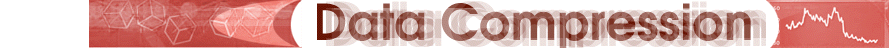Introduction
Algorithm
- DCT
- Coefficient Quantization
- Lossless Compression
Color
Future

Coefficient Quantization

Quantization is the process of reducing the number of bits needed to store an integer value by reducing the precision of the integer. Given a matrix of DCT coefficients, we can generally reduce the precision of the coefficients more and more as we move away from the DC coefficient. This is because the farther away we are from the DC coefficient, the less the element contributes to the graphical image, and therefore, the less we care about maintaining rigorous precision in its value (Nelson 364).

The JPEG algorithm implements quantization using a separate quantization matrix, an example of which is shown below.

A Sample Quantization Matrix

 3 5 7 9 11 13 15 17 5 7 9 11 13 15 17 19 7 9 11 13 15 17 19 21 9 11 13 15 17 19 21 23 11 13 15 17 19 21 23 25 13 15 17 19 21 23 25 27 15 17 19 21 23 25 27 29 17 19 21 23 25 27 29 31

For every element position in the DCT matrix, a corresponding value in the quantization matrix gives a quantum value indicating what the step size is going to be for that element. The coefficients that are most significant to the compressed rendition of the image (those closer to the upper left corner) are encoded with a small step size, while coefficients that are less important (those closer to the lower right corner) are encoded with larger step sizes. The actual formula for quantization is displayed below.From the formula, one can notice the smaller DCT coefficients of high-frequency elements divided by the larger quantum values will most often result in the high-frequency coefficients being rounded down to zero. The sample matrices below show the effects of quantization on a DCT matrix.

DCT Matrix Before Quantization

 92 3 -9 -7 3 -1 0 2 -39 -58 12 17 -2 2 4 2 -84 62 1 -18 3 4 -5 5 -52 -36 -10 14 -10 4 -2 0 -86 -40 49 -7 17 -6 -2 5 -62 65 -12 -2 3 -8 -2 0 -17 14 -36 17 -11 3 3 -1 -54 32 -9 -9 22 0 1 3

DCT Matrix After Dequantization

 90 0 -7 0 0 0 0 0 -35 -56 9 11 0 0 0 0 -84 54 0 -13 0 0 0 0 -45 -33 0 0 0 0 0 0 -77 -39 45 0 0 0 0 0 -52 60 0 0 0 0 0 0 -15 0 -19 0 0 0 0 0 -51 19 0 0 0 0 0 0

The quantization/dequantization cycle has readily apparent effects. The low-frequency elements near the DC coefficient have been modified, but only by small amounts.

The high-frequency areas of the matrix have, for the most part, been reduced to zero, eliminating their effect on the decompressed image. In this sense, insignificant data has been discarded and the image information has been compressed.

At this point, one might wonder how the values in the quantization matrix are selected. An enormous number of schemes could be used to define these values, and the two most common experimental approaches for testing the effectiveness of such schemes are as follows:

• Measure the mathematical error found between an input image and its output image after it has been decompressed, trying to determine an acceptable level of error.
• Simply "eyeball it". Although judge the effect of decompression on the human eye is purely subjective, it may, in some cases, be more credible than mathematical differences in error levels.

Although JPEG allows for the use of any quantization matrix, ISO has done extensive testing and developed a standard set of quantization values that cause impressive degrees of compression.